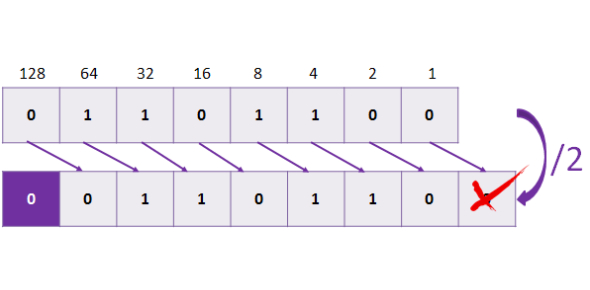# Binary Converter Quiz: Test!

Approved & Edited by ProProfs Editorial Team
At ProProfs Quizzes, our dedicated in-house team of experts takes pride in their work. With a sharp eye for detail, they meticulously review each quiz. This ensures that every quiz, taken by over 100 million users, meets our standards of accuracy, clarity, and engagement.
| Written by Wilbert
W
Wilbert
Community Contributor
Quizzes Created: 1 | Total Attempts: 3,133
Questions: 10 | Attempts: 3,168Settings.

• 1.

### Change the following number to binary (Base Two)   32

• A.

00100000

• B.

00100001

• C.

01000000

• D.

00110000

A. 00100000
Explanation
The given number, 32, is converted to binary by representing each digit in its binary form. In binary, the number 32 is represented as 00100000.

Rate this question:

• 2.

### Change the following number to binary (Base Two) 12

• A.

00001100

• B.

00001010

• C.

10000100

• D.

00000111

A. 00001100
Explanation
The given number is 12. To convert it to binary, we divide the number by 2 repeatedly and note down the remainders. The remainders in reverse order give us the binary representation. In this case, when we divide 12 by 2, we get a quotient of 6 and a remainder of 0. When we divide 6 by 2, we get a quotient of 3 and a remainder of 0. When we divide 3 by 2, we get a quotient of 1 and a remainder of 1. Finally, when we divide 1 by 2, we get a quotient of 0 and a remainder of 1. Therefore, the binary representation of 12 is 00001100.

Rate this question:

• 3.

### Change the following number to binary (Base Two) 74

• A.

00101010

• B.

01100100

• C.

01001010

• D.

01011010

C. 01001010
Explanation
The given correct answer, 01001010, is the binary representation of the number 74. In binary, each digit can only be 0 or 1, and the digits represent increasing powers of 2 from right to left. Starting from the rightmost digit, we have 0*2^0 + 1*2^1 + 0*2^2 + 0*2^3 + 1*2^4 + 0*2^5 + 0*2^6 + 1*2^7, which simplifies to 0 + 2 + 0 + 0 + 16 + 0 + 0 + 128, resulting in 74.

Rate this question:

• 4.

### Change the following number to binary (Base Two) 255

• A.

11101110

• B.

11111111

• C.

01010101

• D.

11111110

B. 11111111
Explanation
The given number, 255, is being converted to binary (Base Two). In binary, each digit can only be 0 or 1. The correct answer, 11111111, represents the binary equivalent of 255.

Rate this question:

• 5.

### Change the following number to binary (Base Two) 100

• A.

01100100

• B.

01101100

• C.

01011001

• D.

01101100

A. 01100100
Explanation
The given number 100 is converted to binary by dividing it by 2 repeatedly and noting the remainders. The remainders are then read in reverse order to obtain the binary representation. In this case, the binary representation of 100 is 01100100.

Rate this question:

• 6.

### Change the following number to binary (Base Two) 128

• A.

10000100

• B.

01000000

• C.

10000000

• D.

11000000

C. 10000000
Explanation
The given number, 128, when converted to binary (Base Two), is represented as 10000000.

Rate this question:

• 7.

### Change the following number to binary (Base Two) 63

• A.

00011111

• B.

00111100

• C.

00111111

• D.

01111110

C. 00111111
Explanation
The given question asks to convert the number 63 into binary (Base Two). The correct answer is 00111111. In binary, each digit represents a power of two, starting from the rightmost digit as 2^0, then 2^1, 2^2, and so on. By adding the corresponding powers of two for each digit in the binary representation, we get the decimal value of the number. In this case, 00111111 represents (0*2^7) + (0*2^6) + (1*2^5) + (1*2^4) + (1*2^3) + (1*2^2) + (1*2^1) + (1*2^0), which simplifies to 63.

Rate this question:

• 8.

### Change the following number to binary (Base Two) 23

• A.

00010111

• B.

00011011

• C.

00010110

• D.

00110111

A. 00010111
Explanation
The correct answer is 00010111 because when converting the decimal number 23 to binary, we divide it by 2 repeatedly and record the remainder. Starting from the rightmost digit, the remainders are 1, 1, 1, 0, 1, 0, 0, 0. These remainders give us the binary representation of 23, which is 00010111.

Rate this question:

• 9.

### Convert 00110110 to its Base Ten number.

54
Explanation
To convert a binary number to its base ten equivalent, each digit is multiplied by 2 raised to the power of its position, starting from the rightmost digit. In this case, the binary number 00110110 has 8 digits. Starting from the rightmost digit, the first digit is 0, the second digit is 1, the third digit is 1, and so on. Multiplying each digit by the corresponding power of 2 and summing them up, we get 0x2^7 + 0x2^6 + 1x2^5 + 1x2^4 + 0x2^3 + 1x2^2 + 1x2^1 + 0x2^0, which simplifies to 0 + 0 + 32 + 16 + 0 + 4 + 2 + 0 = 54.

Rate this question:

• 10.

### Convert 00000101 to its Base Ten number.Back to top